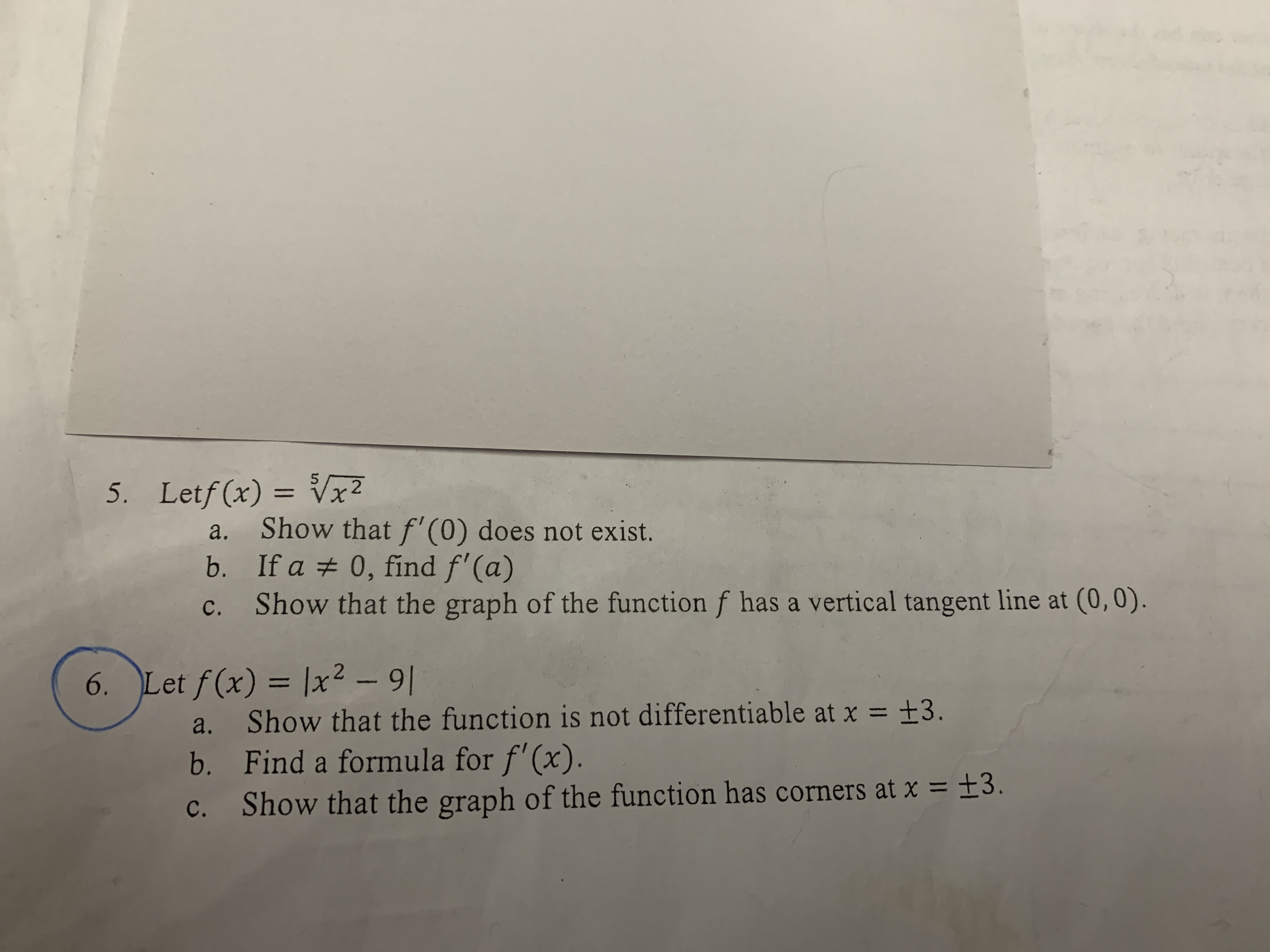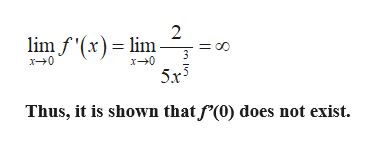# 5. Letf (x) V2Show that f'(0) does not exist.b. If a 0, find f'(a)c. Show that the graph of the function f has a vertical tangent line at (0,0).a.6. Let f (x) = lx2 - 9Show that the function is not differentiable at x = ±3.b. Find a formula for f'(x).c. Show that the graph of the function has corners at x = ±3.a.

Question
49 views

I need help with solving number 5 part a b c. Thank you!help_outlineImage Transcriptionclose5. Letf (x) V2 Show that f'(0) does not exist. b. If a 0, find f'(a) c. Show that the graph of the function f has a vertical tangent line at (0,0). a. 6. Let f (x) = lx2 - 9 Show that the function is not differentiable at x = ±3. b. Find a formula for f'(x). c. Show that the graph of the function has corners at x = ±3. a. fullscreen
check_circle

Step 1

(a)

Consider the given function:

Step 2

Now, differentiate with respect to x:

Step 3

Now, take limit x ten...help_outlineImage Transcriptionclose2 lim f'(xlim 3 x0 x0 Thus, it is shown that f'(0) does not exist. fullscreen

### Want to see the full answer?

See Solution

#### Want to see this answer and more?

Solutions are written by subject experts who are available 24/7. Questions are typically answered within 1 hour.*

See Solution
*Response times may vary by subject and question.
Tagged in

### Derivative